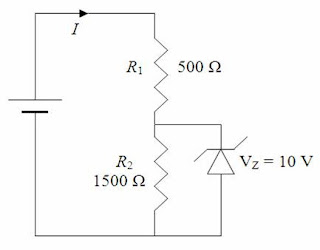## Wednesday, May 18, 2011

### AIEEE 2011 Question (MCQ) on Magnetic Field due to Current Carrying Conductors

Wealth without work

Pleasure without conscience

Science without humanity

Knowledge without character

Politics without principle

Commerce without morality

Worship without sacrifice."

– Mahatma Gandhi

The following multiple choice question included in the All India Engineering/Architecture Entrance Examination (AIEEE) 2011 will be interesting and useful to you:

A current I flows in an infinitely long wire with cross section in the form of a semi-circular ring of radius R. The magnitude of the magnetic induction along its axis is

(1) μ0 I/4πR

(2) μ0 I/π2R

(3) μ0 I/2π2R

It would have been better if the magnitude of the magnetic induction at the axis was asked for since the words along the axis usually means directed along the axis. [The component of field directed along the axis is zero].

Forget about it. The question setter requires you to calculate the magnetic flux density at points on the axis (of the semicircular ring shaped cross section of the infinitely long wire). In the adjoining figure we have shown the cross section (of the given wire) lying in the XY plane. The length of the wire is along the Z-axis and the current in the wire is supposed to flow along the negative Z-direction. The broad infinitely long wire can be imagined to be made of a large number of infinitely long straight wire strips, each of small width dℓ.

With reference to the figure, we have dℓ = Rdθ.

The magnetic flux density due to the above strip is shown as dB1 in the figure. It has an X-component dB1 sinθ and a Y-component dB1cosθ. When we consider a similar strip of the same with dlocated symmetrically with respect to the Y-axis, we obtain a contribution dB2 to the flux density. The flux density dB2 has the same magnitude as dB1. It has X-component dB2 sinθ and Y-component dB2cosθ. The X-components of dB1 and dB1 are of the same magnitude and direction and they add up. But the Y-components of dB1 and dB1 are in opposite directions and have the same magnitude. Therefore they get canceled. The entire conductor therefore produces a resultant magnetic field along the negative X-direction.

The wire strip of width dℓ cn be imagined to be an ordinary thin straight infinitely long wire carrying current Id/πR since the total current I flows through the semicircular cross section of perimeter πR. Putting dB1 = dB2 = dB we have

dB = μ0 (Id/πR)/2πR = μ0 (IRdθ/πR)/2πR = μ0Idθ/2π2R

The X-component of the above field is (μ0I/2π2R) sinθ dθ

The field due to the entire conductor is B = 0π [(μ0I/2π2R) sinθ]dθ

Or, B = μ0I/π2R since 0π sinθ dθ = 2

## Wednesday, May 04, 2011

### Kerala Engineering Entrance (KEAM) 2011 Question (MCQ) on Zener Diode

“Everything should be made as simple as possible, but not simpler”

– Albert Einstein

The following multiple choice question on zener diode was included in Kerala Engineering Entrance (KEAM) 2011 question paper. If you identify that R1 is the current limiting resistor and R2 is the load resistor in the simple shunt voltage regulator making use of a zener diode, you won’t have any confusion. Here is the question:In the circuit given the current through the zener diode is

(a) 10 mA

(b) 6.67 mA

(c) 5 mA

(d) 3.33 mA

(e) 0 mA

Since the zener breakdown voltage (across the zener diode) is 10 V, the voltage across the resistor R2 is 10 V. The current through R2 is 10 V/1500 Ω = 0.00667 A = 6.67 mA.

The voltage drop across R1 is 15 V – 10 V = 5 V.

Therefore, the current through R1 is 5 V/500 Ω = 0.01 A = 10 mA

The above current gets divided between the resistor R2 and the zener diode. Therefore, the current through the zener diode is 10 mA – 6.67 mA = 3.33 mA.

By clicking on the label ‘zener diode’ below this post you can access similar questions on zener diodes posted on this site.